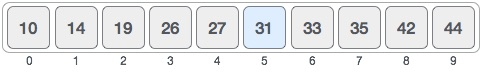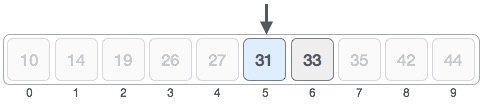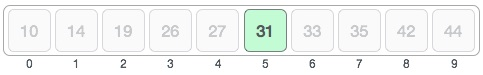## Write C code to implement the Binary Search algorithm

Binary Search can be applied on a sorted array or a list of large size. The time complexity of O(log n) makes it very fast as compared to other searching algorithms. The Binary Search algorithm works on the principle of Divide and Conquer. For this algorithm to work properly, the data collection should be in the sorted form.

Following is an example of a binary search on a sorted array. Let us assume that we are searching for element 31 in the following sorted array using the binary search.First, we need to find half of the array by using the following formula −

` mid = low + (high - low) / 2`

Here it is, 0 + (9 - 0 ) / 2 = 4 . So, 4 is the mid of the array.Now we compare the value stored at location 4, with the value being searched, i.e. 31. We find that the value at location 4 is 27, which is not a match. As the value is greater than 27 and we have a sorted array, so we also know that the target value must be in the upper portion of the array.Now we change our low to mid + 1 and find the new mid-value again.

```low = mid + 1
mid = low + (high - low) / 2```

Our new mid is 7 now. We compare the value stored at location 7 with our target value 31.The value stored at location 7 is not a match, rather it is more than what we are looking for. So, the value must be in the lower part of this location.Hence, we calculate mid again. This time it is 5.We compare the value stored at location 5 with our target value. We find that it is a match.We conclude that the target value 31 is stored at location 5.

Binary search halves the searchable items and thus reduces the count of comparisons to be made to very less numbers.

Here is a C function

```int binarySearch(int arr[], int size, int item) {
int left, right, middle;
left = 0;
right = size - 1;

while (left <= right) {
middle = ((left + right) / 2);

if (item == arr[middle]) {
return (middle);
}
if (item > arr[middle]) {
left = middle + 1;
} else {
right = middle - 1;
}
}

return (-1);
}```

Algorithm C Programming Questions